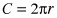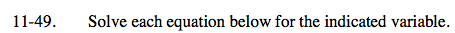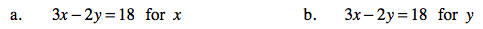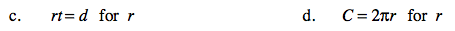Home > AC > Chapter 11 > Lesson 11.1.4 > Problem11-49

11-49.
1. Solve each equation below for the indicated variable. Homework Help ✎

1. 3x − 2y = 18 for x

2. 3x − 2y = 18 for y

3. rt = d for r

4.for rAdd 2y to both sides.
3x = 18 + 2y

Divide both sides by 3.

$x=6+\frac{2}{3}\textit{y}$

See part (a).

$y=\frac{3}{2}x-9$Divide both sides by t.

Divide both sides by 2π.

$r=\frac{C}{2\pi}$